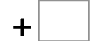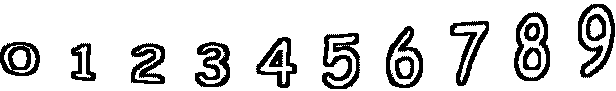27= 56   20= 11654= 115   49= 59   58= 84   73= 100 31= 90   49= 129   41= 120   44= 83